Courses

# Chemistry Test 11 - Equilibrium (I And II), Hydrocarbon, S-Block

## 30 Questions MCQ Test Mock Test Series for JEE Main & Advanced 2022 | Chemistry Test 11 - Equilibrium (I And II), Hydrocarbon, S-Block

Description
This mock test of Chemistry Test 11 - Equilibrium (I And II), Hydrocarbon, S-Block for JEE helps you for every JEE entrance exam. This contains 30 Multiple Choice Questions for JEE Chemistry Test 11 - Equilibrium (I And II), Hydrocarbon, S-Block (mcq) to study with solutions a complete question bank. The solved questions answers in this Chemistry Test 11 - Equilibrium (I And II), Hydrocarbon, S-Block quiz give you a good mix of easy questions and tough questions. JEE students definitely take this Chemistry Test 11 - Equilibrium (I And II), Hydrocarbon, S-Block exercise for a better result in the exam. You can find other Chemistry Test 11 - Equilibrium (I And II), Hydrocarbon, S-Block extra questions, long questions & short questions for JEE on EduRev as well by searching above.
QUESTION: 1

Solution:
QUESTION: 2

Solution:
QUESTION: 3

### Which of the following statements is correct in relation to the halogenation of alkanes ?

Solution:
QUESTION: 4

Which of the following statements regarding sulfur compounds is false?

Solution:
QUESTION: 5
1-Methylcyclohexene is allowed to react with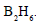The product is then treated with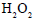and NaOH.
The reaction is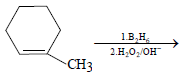The product formed is
Solution:
QUESTION: 6
By which of the following reactions can the conversion of cychlohexene (I) into 1,3-cyclohexadiene (III) be effected
Solution:
QUESTION: 7
When propyne is treated with aqueous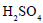in the presence of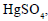the major product formed is
Solution:
QUESTION: 8
Consider the following reaction sequence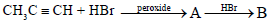The products (A) and (B) are, respectively
Solution:
QUESTION: 9
A hydrocarbon (A) is allowed to react with sodium amide and then with ethyl bromide to produce another hydrocarbon (B) which one ozonolysis produces propanoic acid only. The hydrocarbons (A) and (B) are, respectvely,
Solution:
QUESTION: 10
The nitration of benzene is an example of
Solution:
QUESTION: 11
Chlorination of toluene in the presence of light and heat followed by treatement with aqueous NaOH and subsequently with dilute HCl gives
Solution:
QUESTION: 12
Among the compounds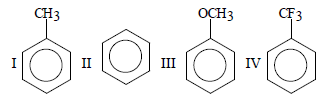the order of decreasing reactivity towards electrophilic substitution is
Solution:
QUESTION: 13
In which of the following reactions is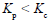?
Solution:
QUESTION: 14

For the equilibrium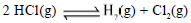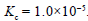If the equilibrium concentration of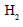and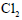is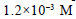and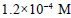respectively then the concentration of HCl is

Solution:
QUESTION: 15
In the reaction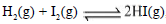the value of the equilibrium constant depends on
Solution:
QUESTION: 16
Which of the following expressions is correct for calculating the degree of dissociation (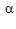) of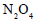(g) (D = vapour density) ?
Solution:
QUESTION: 17

For the equilibrium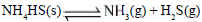Solution:
QUESTION: 18
For the eqilibrium,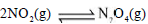14.6 kcal increase in temperature would
Solution:
QUESTION: 19
When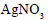is heated in a closed vessel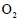gas is liberated and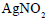is left behind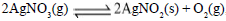,At equilibrium
Solution:
QUESTION: 20
For the heterogeneous equilibrium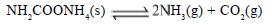the value of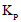is
Solution:
QUESTION: 21
The pH of a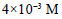HCl solution is
Solution:
QUESTION: 22
Which of the following solvents will undergo selfionization?
Solution:
QUESTION: 23
The solubility product of CuS,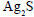and HgS are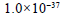,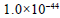and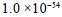respectively. The
solubilities of these suophides are in the order
Solution:
QUESTION: 24
When equal volumes of the following solutions are mixed, the precipitation of AgCl will occur only with (given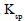of AgCl =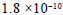)
Solution:
QUESTION: 25
The solubility product of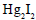is
Solution:
QUESTION: 26
Which of the following is arranged in order of increasing density ?
Solution:
QUESTION: 27
Which of the following alkali-metal hydroxides is the least soluble in water ?
Solution:
QUESTION: 28
Sodium reacts with liquid ammonia to produce
Solution:
QUESTION: 29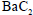reacts with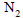to produce
Solution:
QUESTION: 30
Milk of lime is
Solution:

Track your progress, build streaks, highlight & save important lessons and more!

### Similar Content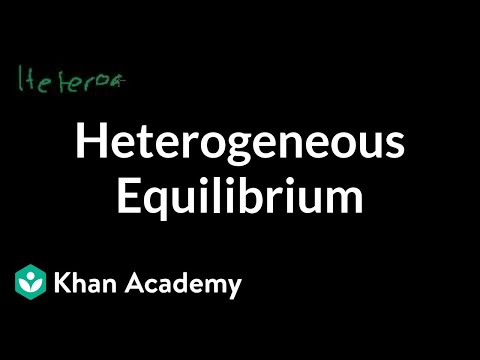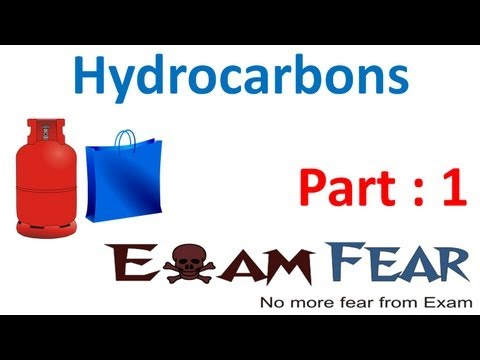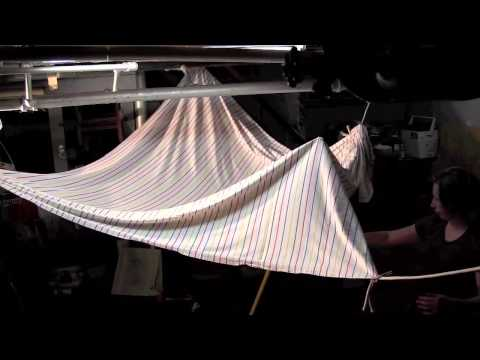### Related tests